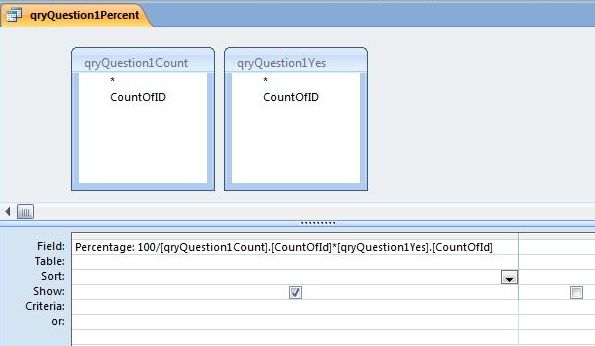## Friday, 2 March 2012

### Calculating Questionnaire Percentages using a Multi-Level Query

Last Saturday I received an interesting question from Ogwang Denis on my Access Tutorial Facebook page. He had created an Access Database to store the results of a survey questionnaire comprised of a number of Yes/No questions, and wanted to know how he could process the answers in a query to produce a percentage.

The solution to this problem is not quite as straight forward as it may at first seem. As Oswang correctly points out, the table field we want to process is of the Yes/No Data Type.  Moreover, each answer to this question is contained in separate interviewee records'.  The task can be articulated into three distinct stages:
1. Count how many interviewees' have been asked this question regardless of answer given.
2. Count how many interviewees' answered YES to this question.
3. Calculate the percentage of Yes answers on the basis of information obtained in stages 1 and 2 above.
Each of these three stages is accomplished with a separate Query.  Moreover, the 3rd stage query uses the summary data provided by the two queries created in the first two stages. As such the final query is multi-levelled - the actual table data represents the first level; the stage 1 and 2 queries, the second; and the third stage query represents the third and final level.  We will see how this multi-levelling is achieved shortly.

Sample Exercise:
Let's look at a simplified database that I created in order to illustrate these stages.  The table, from which the queries are based, contain three questions in each record.  Each question is of the Yes/No Data Type.Figure 1: The sample data we shall be working with is stored in a table called tblQuestionnaire.   Each record represents a separate interviewee's set of answers.  In this exercise we are just interested in their answers' to Question1.
Stage 1:
The first stage involves creating a query to count the number of interviewee records - that is to say, the count of how many times Question1 was asked.  This just requires a single query column based on the count of  the ID field in tblQuestionnaire.  We shall save this query as qryQuestion1Count:Figure 2: The stage one query saved as qryQuestion1Count.
Stage 2:
The second stage involves creating a query to count the number of YES answers to Question1. This is achieved by counting the ID field of tblQuestionnaire as we did in the first query, but this time we shall also add a second query column based on the Question1 field. This column needs to contain a criteria to filter out the YES answers (since -1 represents YES when dealing with the Yes/No Data Type, the criteria will be = -1).  As such we are creating a query to count the ID fields of all records WHERE Question1 = -1. We shall call this query qryQuestion1Yes:Figure 3:  The stage two query saved as qryQuestion1Yes.  This counts the number of ID fields (and hence records) in tblQuestionnaire WHERE Question1 = -1.
Stage 3:
For the third and final stage, we need to create a query based on the previous two queries.  We do this by selecting qryQuestion1Count (the stage one query), and qryQuestion1Yes (the stage two query), from the QUERIES tab of the  SHOW TABLE dialog form, when the Query Design Window opens.Figure 4: The Third Stage Query - qryQuestion1Percent.

The Result:
In our sample data the question was asked 20 times, and was answered YES 10 times. As such, if we work out the calculation manually, the figures are:  100/20 * 10. The answer is 50%, which is indeed the result of the stage three query when it is run.Figure 5: Query result returned by qryQuestion1Percent on sample data.